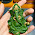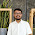## Saturday, 1 November 2014

### Caesar Cipher

```import java.io.*;
public class Caesar_cipher
{
public static void main(String soham[]) throws Exception
{
BufferedReader br = new BufferedReader(new InputStreamReader(System.in));

// enter string to encrypt
System.out.print("\nEnter String to Encrypt: ");
String pt = br.readLine();

// enter key to encrypt
System.out.print("\nEnter key to Encrypt: ");
int key = Integer.parseInt(br.readLine());

// passing plain_text and key to method encrypt
String ct = encrypt(pt,key);

// printing encrypted text
System.out.print("\nEncrypted Text: " + ct);

System.out.println();

// enter key to decrypt the encrypted text
System.out.print("\nEnter key to Decrypt: ");
int dkey = Integer.parseInt(br.readLine());

// comparing encrypted_key and decrypted_key
if(key == dkey)
{
// if match is found passing cipher_text and key to method decrypt
String pt1 = decrypt(ct,key);
System.out.print("\nDecrypted Text: " + pt1);
}
else
{
// if match is not found cipher_text will not be decrypted...
System.out.print("\nNot Authorized...");
}

System.out.println();
}

public static String encrypt(String pt,int key)
{
char c;
int i,num = 0;

StringBuffer sb = new StringBuffer(pt);

int len = sb.length();
for(i = 0; i <= (len-1); i++)
{
num = pt.charAt(i) - 65;
num = num + key;
c = (char)(num+65);
sb.setCharAt(i,c);
}
return new String(sb);
}

public static String decrypt(String ct,int key)
{
char c;
int i,num = 0;

StringBuffer sb = new StringBuffer(ct);

int len = sb.length();
for(i=0;i <= (len-1);i++)
{
num = ct.charAt(i) + 65;
num = num - key;
c = (char)(num-65);
sb.setCharAt(i,c);
}
return new String(sb);
}
}
```
```Output```

#### 3 comments:

1.Hey Soham,

It had been a long time you posted something new. I was eagerly waiting for your next blog and here it is. Its good to see you making programs of algorithms. This might help you a lot in long run. I enjoyed compiling and executing it. Thanks a lot for sharing this with us.

Have a nice day!
Regards,
Rohan Chaubey.

2.Thanks Rohan Chaubey

1.My pleasure! :)